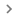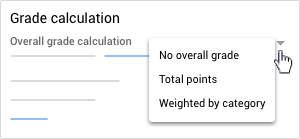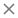For your grading system, you can choose Total points or Weighted by category grading. In both, grades are calculated for you, and you can let students see their overall grade.

If you don’t want to use a grading system, you can choose No overall grade. Grades won’t be calculated and students can’t see an overall grade.

You can also organize classwork with grade categories, such as Essays, Homework, and Tests. For example, if your class has 4 essay assignments, you can organize them in an Essays category.

To have grades calculated for you and an option to let students see their overall grade, use Total points or Weighted by category grading.

An overall grade is calculated for you by dividing the total points a student earns by the total points possible in the class. You can create grade categories and set point values for classwork in each category. For example:
 Essay category Test category Student points/Points possible Overall grade Essay 1: 90/100 Test 1: 95/100 185/200 92.5%
The table shows that a student scored 90 points out of 100 on an essay, and 95 points out of 100 on a test. The student earned a total of 185 points out of 200 possible in the class. Divided, this gives an overall grade of 92.5%.

You assign weights (percentages) to grade categories, and an overall grade is calculated for you. This system calculates as follows:
1. Finds a student's average for a category.
2. Multiplies each category’s average by its weight (as a decimal), giving category scores.
3. Adds the category scores for an overall grade out of 100%.
 Essay category:  50% of grade Test category: 50% of grade Overall grade out of 100% Essay 1: 70/100 Test 1: 90/100 Essay 2: 80/100 Test 2: 100/100 (70 + 80) ÷ 2 = 75 avg.  75 x .5 = 37.5 (90 + 100) ÷ 2 = 95 avg. 95 x .5 = 47.5 Category score = 37.5% Category score = 47.5% Overall grade: 37.5 + 47.5 = 85%

The table shows weighted by category grading. In the Essay category, the student averaged 75. Multiplied by the category weight, .5, this gives 37.5% for Essays. In the Test category, the student averaged 95. Multiplied by the category weight, .5, this gives 47.5% for Tests. Adding the category scores (37.5 + 47.5) gives the student an overall grade of 85%.

## Set up a grading system

You can only select a grading system in the web version of Classroom.

2. Click your classSettings.3. Next to Overall grade calculation, select one:
• Total points—Divides total points earned by total points possible. You can let students see an overall grade.
• Weighted by category—Adds the scores across categories. You can let students see an overall grade.4. (Optional) To make the overall grade visible to students on their profile page, click Show.
Note: This option isn’t available when grade calculation is set to No overall grade.5. In the top-right corner, click Save.

For these options, you assign default point values to the grade categories you create.

Note: You can only create grade categories in the web version of Classroom.

2. Click your classSettings.6. Under Default points, enter a whole number.8. In the top-right corner, click Save.

For this option, you assign percentages to the categories you create.

Note: You can only create grade categories in the web version of Classroom.

2. Click your classSettings.3. Next to Overall grade calculation, select Weighted by category from the menu.6. Under Percentage, enter a whole number.8. In the top-right corner, click Save.
Note: To save your settings, the categories must add up to 100%.

• Removes the grade category from the corresponding classwork.
• Doesn't delete the classwork itself.
2. Click your classSettings.3. Next to the grade category you want to remove, click Remove.